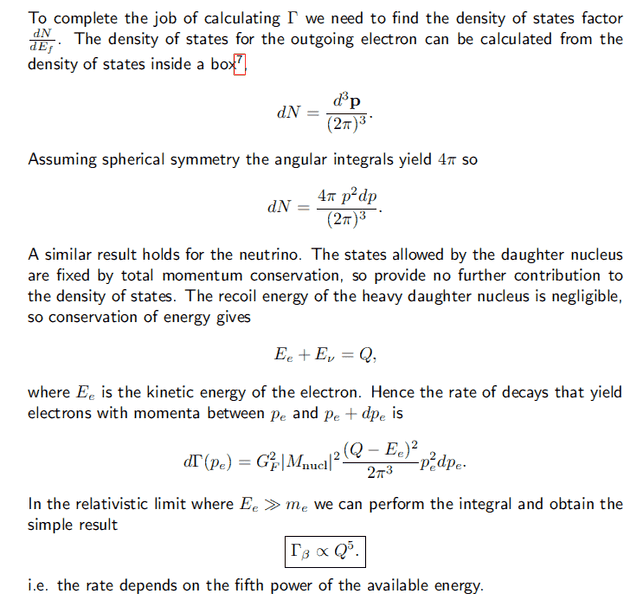# Fermi Golden Rule - Beta Decay

• unscientific

#### unscientific

We consider the following beta decay:

$$^A_ZX \rightarrow ^A_{Z+1} Y + e^{-} + \nu_e$$

The Fermi golden rule is given by:

$$\Gamma = \frac{2\pi}{\hbar} |A_{fi}|^2 \frac{dN}{dE_f}$$

Reaction amplitude is given by ##A_{fi} = G_F M_{nucl} ## while density of states is given by ##dN = \frac{4 \pi p^2 dp}{(2\pi)^3} ## and ##\frac{dp}{dE} = \frac{1}{2}##.

Putting all these together, it gives :

$$\Gamma = \frac{2\pi}{\hbar} G_p^2M_{nucl}^2 \frac{p^2}{2\pi^2} \frac{1}{2} = \frac{\pi}{2\hbar}G_p^2 M_{nucl}^2 p^2$$

$$d\Gamma = \frac{\pi}{\hbar}G_p^2 M_{nucl}^2 p~\ dp$$

I'm not sure how the text derived this.There are two mistakes in your calculation:

1) You are forgetting the neutrino phase space ##\frac{d^3p_\nu}{(2\pi)^3}##
2) It is not true that ##dp/dE=1/2##. In fact, ##p=\sqrt{E^2-m^2}## and hence ##dp/dE=E/p \Rightarrow pdp=EdE##. This is often a useful relation to keep in mind.

Then you have to do the following. Consider the phase space element for both electron and neutrino:
$$dN=\frac{d^3p_e}{(2\pi)^3}\frac{d^3p_\nu}{(2\pi)^3}=\frac{4\pi p_e^2dp_e}{(2\pi)^3}\frac{4\pi p_\nu^2dp_\nu}{(2\pi)^3}=\frac{4\pi p_e^2dp_e}{(2\pi)^3}\frac{4\pi E_\nu^2dE_\nu}{(2\pi)^3},$$
where I considered the neutrino as massless (##p_\nu=E_\nu##). Integrating out the energy of the neutrino you are left with:
$$dN=\frac{4}{(2\pi)^4}E_\nu^2p_e^2dp_e=\frac{4}{(2\pi)^4}(Q-E_e)^2p_e^2dp_e.$$
Therefore:
$$d\Gamma=2\pi G_F^2\left|M_\text{nucl}\right|^2\frac{4}{(2\pi)^4}(Q-E_e)^2p_e^2dp_e=\frac{G_F^2}{2\pi^3}\left|M_\text{nucl}\right|^2(Q-E_e)^2p_e^2dp_e.$$
Which is the result you are looking for. Note that I used ##\hbar=1##.
I hope this is what you were looking for.

•unscientific
There are two mistakes in your calculation:

1) You are forgetting the neutrino phase space ##\frac{d^3p_\nu}{(2\pi)^3}##
2) It is not true that ##dp/dE=1/2##. In fact, ##p=\sqrt{E^2-m^2}## and hence ##dp/dE=E/p \Rightarrow pdp=EdE##. This is often a useful relation to keep in mind.

Then you have to do the following. Consider the phase space element for both electron and neutrino:
$$dN=\frac{d^3p_e}{(2\pi)^3}\frac{d^3p_\nu}{(2\pi)^3}=\frac{4\pi p_e^2dp_e}{(2\pi)^3}\frac{4\pi p_\nu^2dp_\nu}{(2\pi)^3}=\frac{4\pi p_e^2dp_e}{(2\pi)^3}\frac{4\pi E_\nu^2dE_\nu}{(2\pi)^3},$$
where I considered the neutrino as massless (##p_\nu=E_\nu##). Integrating out the energy of the neutrino you are left with:
$$dN=\frac{4}{(2\pi)^4}E_\nu^2p_e^2dp_e=\frac{4}{(2\pi)^4}(Q-E_e)^2p_e^2dp_e.$$
Therefore:
$$d\Gamma=2\pi G_F^2\left|M_\text{nucl}\right|^2\frac{4}{(2\pi)^4}(Q-E_e)^2p_e^2dp_e=\frac{G_F^2}{2\pi^3}\left|M_\text{nucl}\right|^2(Q-E_e)^2p_e^2dp_e.$$
Which is the result you are looking for. Note that I used ##\hbar=1##.
I hope this is what you were looking for.

Brilliant, thank you! It's people like you that make physics forums a great resource for everyone.

•Einj
Integrating out the energy of the neutrino you are left with:
$$dN=\frac{4}{(2\pi)^4}E_\nu^2p_e^2dp_e=\frac{4}{(2\pi)^4}(Q-E_e)^2p_e^2dp_e.$$

Which is the result you are looking for. Note that I used ##\hbar=1##.
I hope this is what you were looking for.

Just a quick question: why is ##dE_v = 1## ?

No, it's not one. I simply integrated over it. If you want to be rigorous I should have written ##d^2N=f(E_\nu,p_e)dp_edp_\nu##, i.e. a double differential. In the second step I integrated over the energy of the neutrino and so I arrived to a single differential.

No, it's not one. I simply integrated over it. If you want to be rigorous I should have written ##d^2N=f(E_\nu,p_e)dp_edp_\nu##, i.e. a double differential. In the second step I integrated over the energy of the neutrino and so I arrived to a single differential.

Then won't the result become: ## \int E_v^2 dE_v = \frac{1}{3} E_v^3##?

Sorry, maybe I've been a little bit too implicit. Inside the matrix element you always have a delta function for conservation of energy. Therefore you have should have something like:
$$\int dE_\nu E_\nu^2\delta(E_\nu+E_e-Q)=(Q-E_e)^2.$$
Is it clear? Sorry for being obscure :P

•unscientific
Sorry, maybe I've been a little bit too implicit. Inside the matrix element you always have a delta function for conservation of energy. Therefore you have should have something like:
$$\int dE_\nu E_\nu^2\delta(E_\nu+E_e-Q)=(Q-E_e)^2.$$
Is it clear? Sorry for being obscure :P

Got it, thanks!